Next: The Problem Space Up: Grammatical Evolution Previous: Grammatical Evolution

## Example Individual

Consider an individual, see Figure 2, made up of the following genes (expressed in decimal for clarity). Figure 2

These numbers will be used to look up the table in Section 1 which describes the BNF grammar for this particular problem. To complete the BNF definition for a C function, we need to include the following rules with the earlier definition:

<func> ::= <header>

<header> ::= float symb(float X) { <body> }

<body> ::= <declarations><code><return>

<declarations ::= float a;

<code> ::= a = <expr>;

<return> ::= return (a);


The first few rules don't involve any choice, so all individuals are of the form:

float symb(float x)
{
a = <expr>;
return(a);
}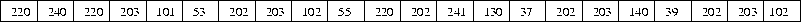Concentrating on the <expr> part, we can see that there are four productions to choose from. To make this choice, we read the first gene from the chromosome, and use it to generate a protein in the form of a number. This number will then be used to decide which production rule to use, thus we have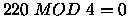which means we must take the first production, namely, 1A. We now have the following

<expr> <op> <expr>


Notice that if this individual is subsequently wrapped, the first gene will still produce the protein 220. However, depending on previous proteins, we may well be examining the production of another rule, possibly with a different amount of choices. In this way, although we have the same protein it results in a different physical trait.

Continuing with the first <expr>, a similar choice must be made, again using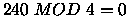, that is 1A. We now have the following

<expr> <op> <expr> <op> <expr>


Similarly again we have the same choice for the first <expr>, the result being

<expr> <op> <expr> <op> <expr> <op> <expr> <op> <expr>


Now the first <expr> will be determined by the gene value 203 which gives us rule 1D which is <var>. The next gene then determines what value <var> shall take,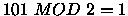i.e. rule 4B, which turns out to be 1.0. We now have the following

1.0 <op> <expr> <op> <expr> <op> <expr> <op> <expr>


The next gene will determine what <op> will become, so we have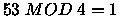, which gives a -. The next <expr> has then to be expanded using the gene value 202, that is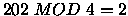. So we now have

1.0 - <pre-op><expr> <op> <expr> <op> <expr> <op> <expr>


There can only be one outcome for a <pre-op> that being Sin, therefore, no decision has to be made and so no gene is read.
The next <expr> is then expanded by the value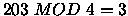which is rule 1D, or <var> . It's value is then determined by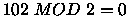, rule 4A, and the resulting expression is

1.0 - Sin(x) <op> <expr> <op> <expr> <op> <expr>


The mapping continues until eventually, we are left with the following expression:

1.0 - Sin(x)*Sin(x) - Sin(x)*Sin(x)


Notice how all of the genes were required in this case, had there been any extra genes they would have been simply ignored.Next: The Problem Space Up: Grammatical Evolution Previous: Grammatical Evolution
root
1998-10-02